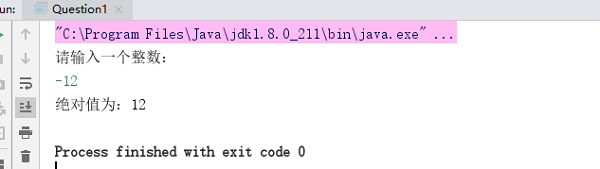# java输出一个值的绝对值怎么编写？

TheDisguiser 2020-07-07 22:12:10 java常见问答 7635

```import java.util.Scanner;
public class Question1
{
public static void main(String[] args)
{
//从键盘得到一个值
Scanner sc = new Scanner(System.in); //声明一个标准输入扫描器
System.out.println("请输入一个整数：");
int n = sc.nextInt(); //得到整数
sc.close(); //关闭扫描器
//调用转换为绝对值的函数
n = ToAbs(n);
System.out.println("绝对值为：" + n);
}
//取绝对值方法
//int类型存入是以二进制补码的形式在内存中，要取绝对值，只需要改变其符号位
public static int ToAbs(int n)
{
int i = n >> 31; //int 为32位，右移31位得到符号位，赋值给i，若为正，则i==0;负，i==-1
return ((n ^ i) - i); //一个数^0==原数，数^-1==数的绝对值-1 ==》 绝对值=负数异或-1取反+1
}
}``````public class 绝对值
{
public static void main(String[] args)
{
System.out.println("输入一个整数n:\n");
Scanner s = new Scanner(System.in);
int n = s.nextInt();
if (n >= 0)
{
n = n;
}
else
{
n = -1 * n;
}
System.out.println(n);
}
}```

java输入一个数求绝对值，java绝对值怎么写?